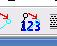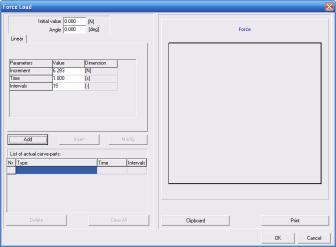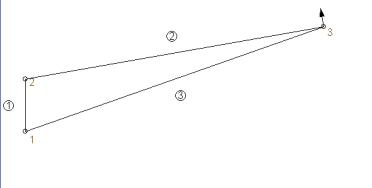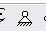Stress and Strain in a wire braced wing.

This continues my Solar plane design saga.

My proposed wire braced wing….To analyze the loads my first attempt was to do it algebraically summing all the forces and torques and solving the resultant simultaneous equations. After spending a whole evening with excel and many mages of have erased vector diagrams, I gave up at 1 in the morning and went to bed.  The next morning I got up and tried to find some software…

I found a cool program that satisfies my needs in demo mode.  In is SAM 5 at http://www.artas.nl/  It was designed to analize linkages and kinematics, so I had to twist it a bit to satisfy my needs.

2)Plan out how to model your geometry…. (I made several attempts, I’m only going to show the one that worked.)

3)Enter the system in SAM.

3a)Hit the file new… enter your workspace size…3b)Choose the linksmenu item and place you geometry in the right half window

The geometry has been roughly entered…Notice how it has labeled the 3 elements or bars…

Now to make the geometry dimensionally correct…

hit the point/numbers toolbar3c) Now highlight the points and enter the coordinates… (in mm)

My points….

Point 2 (0,0)

Point 1 (0,-210)

Point 3 (1201,212) I calculated this from the 10 degree half dihedral angle, you could also get it from your CAD program…

4)After the geometry is entered we need to enter the forces….

My fuselage, motor,telemetry etc.. mass 1800 gm.

Design load 6 g  so 1.8Kg * 9.8 (g) * 6 =106 Newtons force.

Each wing only does half or 53 newtons…

Since the wing is tilted 10 degrees the force is actually 53/ Cos(10) = 54 newtons.

The force is at an angle of 100 degrees from horizontal, ie 10 degrees tipped in from vertical 90 degrees.

Where does this force act? I assumed it acts at half span a pessimistic assumption…

Since SAM won’t let me put a force at the half span position I have two choices

Put the force at the strut attach point (even more pessimistic point 3 in the drawing) or put in two forces at points 2,3 that sum to the proper force and torque.  I did it both ways but for this tutorial I’m going to keep it simple.

4a)Go to the menu and select  Loads -> force Then click on point 3

This brings up this dialog:After I entered my force info and hit add:I entered a force of 54 newtons and 100 degrees, not incrementing and

Time of 1 sec with 1 interval. (This was done to trick the program that is trying to model dynamic behavior into calculating fixed loads) Then hit add and ok….

When this is done the diagram looks like:5)Make the program happy….

I needed to do a couple of things to get the program to run my simulation.

First I anchored my fuselage…. Hit thetool bar and anchor point #16)Do the analysis…. If you just tell it to analize at this point it complains that no input is defined. Since the program wants to do dynamic movement I had to enter a movement.

I choose to rotate around point 1.

So menu input motion-> angle then click on point 1 and 2 giving this dialog:Note that the Time and intervals entered here MUST match the time and intervals entered in the force dialog… Hit add -> ok and the picture now looks like:Notice that there is a graph, but no data……. We forgot to ask for data so click on theanalize tool bar  giving this dialog:Hit element Data and then click on the bar #3  giving the dialog:Tell it you want the normal force…. And repeat for all three bars, 1,2,3

This now give you the results in the graph….

I want to do one more thing before I show the results…. In the analize dialog click on Node data and then point #1 giving the dialog:I asked for the X and y Force components at point #1.

One last change….. I can’t do gravity in English units….

So I entered everything in Si/Metric units… but the program is quite helpful…

I wanted to see the forces displayed in pounds force  so

Then click on Setup Units and Dimensions….

Select display force in pounds force and we get the following data…This says :

The center post is under 12 lbs of compression,

The wing spar is under 73 pounds of compression

The wire is under 74 pounds of tension.

The Whole thing is lifting 11.95 pounds

Reality check… 11.95*2 (for both wings) = 24 lbs /6 G = 4 pounds ~= 1800 gm